```1 2 3 4 5``` ``````system.time({ install.packages(c("BiocManager", "scales", "viridis", "ggsci")) BiocManager::install(c("slingshot", "BUSpaRse", "SingleR", "DropletUtils", "scater", "scran", "tradeSeq"), Ncpus = 2) }) ``````
``````Installing packages into ‘/usr/local/lib/R/site-library’
(as ‘lib’ is unspecified)

also installing the dependency ‘gridExtra’

Bioconductor version 3.10 (BiocManager 1.30.10), R 3.6.2 (2019-12-12)

Installing package(s) 'BiocVersion', 'slingshot', 'BUSpaRse', 'SingleR',

also installing the dependencies ‘bit’, ‘formatR’, ‘bibtex’, ‘iterators’, ‘annotate’, ‘bit64’, ‘blob’, ‘zlibbioc’, ‘bitops’, ‘Rhtslib’, ‘lambda.r’, ‘futile.options’, ‘interactiveDisplayBase’, ‘pkgmaker’, ‘registry’, ‘rngtools’, ‘gridBase’, ‘foreach’, ‘doParallel’, ‘RcppEigen’, ‘softImpute’, ‘genefilter’, ‘ade4’, ‘rncl’, ‘RNeXML’, ‘Biobase’, ‘IRanges’, ‘RSQLite’, ‘XML’, ‘BiocFileCache’, ‘rappdirs’, ‘XVector’, ‘rtracklayer’, ‘Rsamtools’, ‘ProtGenerics’, ‘RCurl’, ‘GenomeInfoDbData’, ‘GenomicAlignments’, ‘RcppAnnoy’, ‘RcppHNSW’, ‘futile.logger’, ‘snow’, ‘AnnotationHub’, ‘locfit’, ‘R.oo’, ‘R.methodsS3’, ‘sitmo’, ‘beeswarm’, ‘vipor’, ‘irlba’, ‘rsvd’, ‘NMF’, ‘howmany’, ‘locfdr’, ‘RSpectra’, ‘kernlab’, ‘zinbwave’, ‘phylobase’, ‘pracma’, ‘princurve’, ‘ape’, ‘igraph’, ‘matrixStats’, ‘SingleCellExperiment’, ‘SummarizedExperiment’, ‘AnnotationDbi’, ‘AnnotationFilter’, ‘biomaRt’, ‘Biostrings’, ‘BSgenome’, ‘data.table’, ‘ensembldb’, ‘GenomeInfoDb’, ‘GenomicFeatures’, ‘GenomicRanges’, ‘plyranges’, ‘RcppParallel’, ‘S4Vectors’, ‘zeallot’, ‘RcppArmadillo’, ‘RcppProgress’, ‘DelayedArray’, ‘DelayedMatrixStats’, ‘BiocNeighbors’, ‘BiocParallel’, ‘ExperimentHub’, ‘beachmat’, ‘edgeR’, ‘rhdf5’, ‘HDF5Array’, ‘R.utils’, ‘dqrng’, ‘Rhdf5lib’, ‘BiocGenerics’, ‘ggbeeswarm’, ‘BiocSingular’, ‘limma’, ‘statmod’, ‘clusterExperiment’, ‘pbapply’

Old packages: 'curl', 'dplyr', 'DT', 'farver', 'jsonlite', 'mime', 'rlang',
'rprojroot', 'xfun', 'xtable', 'yaml'

user   system  elapsed
3927.901  271.269 2157.764
``````
 `1` ``````devtools::install_github('cole-trapnell-lab/leidenbase') ``````
``````Downloading GitHub repo cole-trapnell-lab/leidenbase@master

[32m✔[39m  [90mchecking for file ‘/tmp/Rtmp3ZgCkF/remotes82750bfffa/cole-trapnell-lab-leidenbase-c22a7d0/DESCRIPTION’[39m[36m[36m (345ms)[36m[39m
[90m─[39m[90m  [39m[90mpreparing ‘leidenbase’:[39m[36m[39m
[32m✔[39m  [90mchecking DESCRIPTION meta-information[39m[36m[39m
[90m─[39m[90m  [39m[90mcleaning src[39m[36m[39m
[90m─[39m[90m  [39m[90mchecking for LF line-endings in source and make files and shell scripts[39m[36m[39m
[90m─[39m[90m  [39m[90mchecking for empty or unneeded directories[39m[36m[36m (578ms)[36m[39m
[90m─[39m[90m  [39m[90mbuilding ‘leidenbase_0.1.0.tar.gz’[39m[36m[39m

Installing package into '/usr/local/lib/R/site-library'
(as 'lib' is unspecified)
``````
 `1` ``````system("pip3 install kb-python") ``````
 ``` 1 2 3 4 5 6 7 8 9 10 11 12 13 14 15``` ``````library(slingshot) library(BUSpaRse) library(DropletUtils) library(SingleR) library(tidyverse) library(Seurat) library(ggsci) library(viridis) library(Matrix) library(scater) library(scran) library(tradeSeq) library(BiocParallel) library(clusterExperiment) theme_set(theme_bw()) ``````
``````tradeSeq has been updated to accommodate singleCellExperiment objects as output, making it much more memory efficient. Please check the news file and the updated vignette for details.
``````
 ```1 2 3 4 5 6``` ``````# Download data if (!dir.exists("./data")) dir.create("./data") if (!file.exists("./data/neuron_1k_v3_fastqs.tar")) { download.file("http://cf.10xgenomics.com/samples/cell-exp/3.0.0/neuron_1k_v3/neuron_1k_v3_fastqs.tar", "./data/neuron_1k_v3_fastqs.tar", method = "wget", quiet = TRUE) } ``````
 `1` ``````untar("./data/neuron_1k_v3_fastqs.tar", exdir = "./data") ``````
 `1` ``````system("kb ref -d human -i index.idx -g t2g.txt -f1 transcriptome.fasta",intern=TRUE) ``````
 `1` ``````system("chmod -R 777 data/") ``````
 ```1 2 3``` ``````fn <- list.files("data/neuron_1k_v3_fastqs", full.names = TRUE) fn <- fn[str_detect(fn, "R\\d_")] fn ``````
1. 'data/neuron_1k_v3_fastqs/neuron_1k_v3_S1_L001_R1_001.fastq.gz'
2. 'data/neuron_1k_v3_fastqs/neuron_1k_v3_S1_L001_R2_001.fastq.gz'
3. 'data/neuron_1k_v3_fastqs/neuron_1k_v3_S1_L002_R1_001.fastq.gz'
4. 'data/neuron_1k_v3_fastqs/neuron_1k_v3_S1_L002_R2_001.fastq.gz'
 ```1 2``` ``````system(paste("kb count -i reference/mm_tr_index99.idx -g reference/tr2g_mm99.tsv -x 10xv3 -o output", paste(fn, collapse = " ")), intern = TRUE) ``````
 `1` ``````res_mat <- read_count_output("./output/counts_unfiltered", name = "cells_x_genes", tcc = FALSE) ``````
 `1` ``````dim(res_mat) ``````
1. 36711
2. 399524
 ```1 2``` ``````tot_counts <- Matrix::colSums(res_mat) summary(tot_counts) ``````
``````     Min.   1st Qu.    Median      Mean   3rd Qu.      Max.
0.00      1.00      1.00     40.47      5.00 154216.00
``````
 ```1 2``` ``````# Compute barcode rank bc_rank <- barcodeRanks(res_mat, lower = 1000) ``````
 ``` 1 2 3 4 5 6 7 8 9 10 11 12 13 14 15 16 17 18 19 20 21 22 23 24 25``` ``````#' Knee plot for filtering empty droplets #' #' Visualizes the inflection point to filter empty droplets. This function plots #' different datasets with a different color. Facets can be added after calling #' this function with `facet_*` functions. #' #' @param bc_rank A `DataFrame` output from `DropletUtil::barcodeRanks`. #' @return A ggplot2 object. knee_plot <- function(bc_rank) { knee_plt <- tibble(rank = bc_rank[["rank"]], total = bc_rank[["total"]]) %>% distinct() %>% dplyr::filter(total > 0) annot <- tibble(inflection = metadata(bc_rank)[["inflection"]], rank_cutoff = max(bc_rank\$rank[bc_rank\$total > metadata(bc_rank)[["inflection"]]])) p <- ggplot(knee_plt, aes(rank, total)) + geom_line() + geom_hline(aes(yintercept = inflection), data = annot, linetype = 2) + geom_vline(aes(xintercept = rank_cutoff), data = annot, linetype = 2) + scale_x_log10() + scale_y_log10() + annotation_logticks() + labs(x = "Rank", y = "Total UMIs") return(p) } ``````
 ```1 2``` ``````options(repr.plot.width=9, repr.plot.height=6) knee_plot(bc_rank) ``````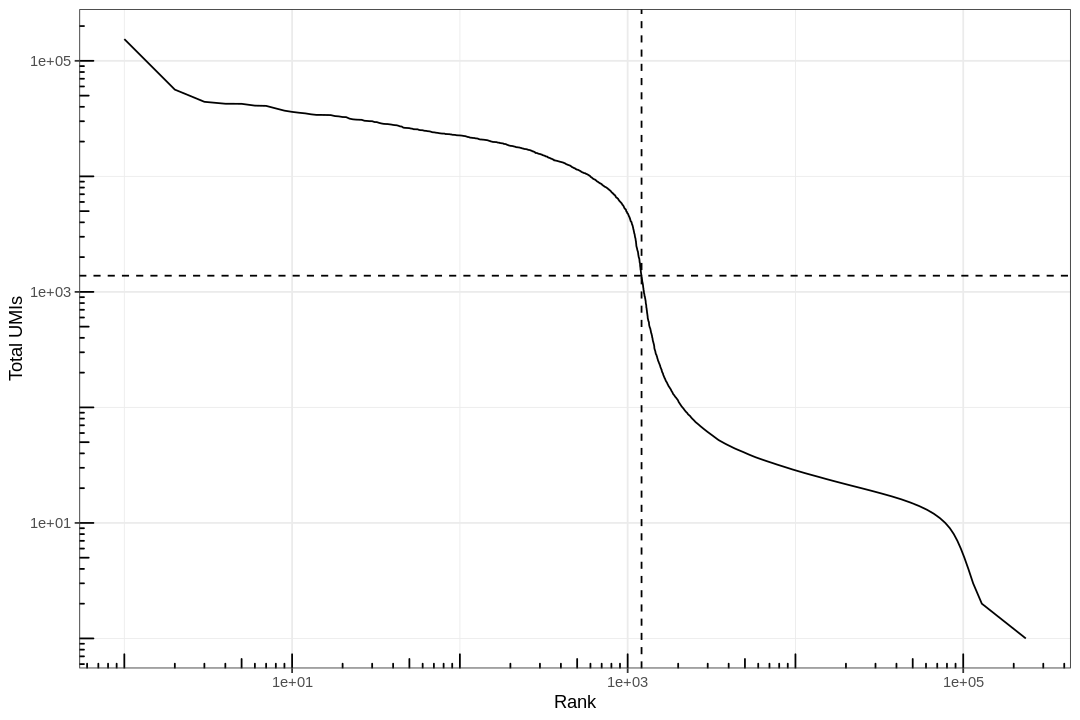```1 2 3 4``` ``````# Filter the matrix res_mat <- res_mat[, tot_counts > metadata(bc_rank)\$inflection] res_mat <- res_mat[Matrix::rowSums(res_mat) > 0,] dim(res_mat) ``````
1. 20198
2. 1210
 `1` ``````rownames(res_mat) <- str_remove(rownames(res_mat), "\\.\\d+") ``````
 ```1 2 3``` ``````mouse.rnaseq <- MouseRNAseqData(ensembl = TRUE) sce <- SingleCellExperiment(assays = list(counts = res_mat)) sce <- logNormCounts(sce) ``````
``````Using temporary cache /tmp/Rtmp3ZgCkF/BiocFileCache

snapshotDate(): 2019-10-22

Using temporary cache /tmp/Rtmp3ZgCkF/BiocFileCache

see ?SingleR and browseVignettes('SingleR') for documentation

Using temporary cache /tmp/Rtmp3ZgCkF/BiocFileCache

Using temporary cache /tmp/Rtmp3ZgCkF/BiocFileCache

Using temporary cache /tmp/Rtmp3ZgCkF/BiocFileCache

Using temporary cache /tmp/Rtmp3ZgCkF/BiocFileCache

Using temporary cache /tmp/Rtmp3ZgCkF/BiocFileCache

Using temporary cache /tmp/Rtmp3ZgCkF/BiocFileCache

see ?SingleR and browseVignettes('SingleR') for documentation

Using temporary cache /tmp/Rtmp3ZgCkF/BiocFileCache

Using temporary cache /tmp/Rtmp3ZgCkF/BiocFileCache

Using temporary cache /tmp/Rtmp3ZgCkF/BiocFileCache

Using temporary cache /tmp/Rtmp3ZgCkF/BiocFileCache

Using temporary cache /tmp/Rtmp3ZgCkF/BiocFileCache

Using temporary cache /tmp/Rtmp3ZgCkF/BiocFileCache

Using temporary cache /tmp/Rtmp3ZgCkF/BiocFileCache

snapshotDate(): 2019-10-29

Using temporary cache /tmp/Rtmp3ZgCkF/BiocFileCache

Using temporary cache /tmp/Rtmp3ZgCkF/BiocFileCache

Using temporary cache /tmp/Rtmp3ZgCkF/BiocFileCache

Using temporary cache /tmp/Rtmp3ZgCkF/BiocFileCache

Using temporary cache /tmp/Rtmp3ZgCkF/BiocFileCache

Using temporary cache /tmp/Rtmp3ZgCkF/BiocFileCache

Using temporary cache /tmp/Rtmp3ZgCkF/BiocFileCache

Warning message:
"Unable to map 2180 of 21214 requested IDs."
``````
 ```1 2``` ``````annots <- SingleR(sce, ref = mouse.rnaseq, labels = colData(mouse.rnaseq)\$label.fine, de.method = "wilcox", method = "single", BPPARAM = MulticoreParam(2)) ``````
 `1` ``````annots ``````
``````DataFrame with 1210 rows and 5 columns
scores
<matrix>
AAACGCTGTAATGTGA    0.0601901483760862:0.242986808015721:0.177660752587073:...
AAACGCTGTCCTGGGT      0.11153546550616:0.321858508786419:0.206383027494298:...
AAAGAACCAGGACATG    0.0585569289411892:0.225038532259566:0.177381655702582:...
AAAGGTACACACGGTC       0.137923692580242:0.3506611435018:0.281933499704427:...
AAAGGTATCACCATAG -0.00511350381259525:0.166880227412372:0.0975899954539976:...
...                                                                        ...
TTTGACTTCGTTCAGA     0.100933655338997:0.327948884202836:0.262011719832591:...
TTTGATCTCCATAGGT     0.0656536744266451:0.280702755051776:0.23375860874247:...
TTTGGAGAGGCTAACG    0.0681422805975054:0.234772340818692:0.176292333613646:...
TTTGGTTAGTAATCCC     0.125780004617712:0.315213344287222:0.258722018820061:...
TTTGTTGGTATGGAAT    0.0512710432754663:0.231028330364938:0.177598025280379:...
first.labels                        tuning.scores      labels
<character>                          <DataFrame> <character>
AAACGCTGTAATGTGA        aNSCs  0.233804530306167:0.219682141602258       aNSCs
AAACGCTGTCCTGGGT         NPCs                                  1:1       aNSCs
AAAGAACCAGGACATG      Neurons                                  1:1       aNSCs
AAAGGTACACACGGTC         NPCs                                  1:1       aNSCs
AAAGGTATCACCATAG         NPCs                              0.5:0.5       aNSCs
...                       ...                                  ...         ...
TTTGACTTCGTTCAGA      Neurons                                  1:1       aNSCs
TTTGATCTCCATAGGT      Neurons                              0.5:0.5       aNSCs
TTTGGAGAGGCTAACG        aNSCs 0.209611614011623:0.0580258853185659     Neurons
TTTGGTTAGTAATCCC      Neurons 0.187703782257732:-0.195976600339204     Neurons
TTTGTTGGTATGGAAT         OPCs 0.299839391397521:-0.199361864824359     Neurons
pruned.labels
<character>
AAACGCTGTAATGTGA         aNSCs
AAACGCTGTCCTGGGT         aNSCs
AAAGAACCAGGACATG         aNSCs
AAAGGTACACACGGTC         aNSCs
AAAGGTATCACCATAG         aNSCs
...                        ...
TTTGACTTCGTTCAGA         aNSCs
TTTGATCTCCATAGGT         aNSCs
TTTGGAGAGGCTAACG       Neurons
TTTGGTTAGTAATCCC       Neurons
TTTGTTGGTATGGAAT       Neurons
``````
 ```1 2 3 4 5 6``` ``````inds <- annots\$pruned.labels %in% c("NPCs", "Neurons", "OPCs", "Oligodendrocytes", "qNSCs", "aNSCs", "Astrocytes", "Ependymal") # Only keep these cell types cells_use <- row.names(annots)[inds] sce <- sce[, cells_use] sce\$cell_type <- annots\$pruned.labels[inds] ``````

# QC¶

 ```1 2 3``` ``````df <- perCellQCMetrics(sce) colData(sce) <- cbind(colData(sce), df) colData(sce) ``````
``````DataFrame with 1146 rows and 8 columns
cell_type       sum  detected   percent_top_50
<character> <numeric> <integer>        <numeric>
AAACGCTGTAATGTGA       aNSCs      7072      3256 19.0328054298643
AAACGCTGTCCTGGGT       aNSCs      9458      4101 12.8991330090928
AAAGAACCAGGACATG       aNSCs      5338      2577 20.7006369426752
AAAGGTACACACGGTC       aNSCs     16892      5111 18.2098034572579
AAAGGTATCACCATAG       aNSCs      1397       882 24.9105225483178
...                      ...       ...       ...              ...
TTTGACTTCGTTCAGA       aNSCs     26203      6091 21.4021295271534
TTTGATCTCCATAGGT       aNSCs      8120      3625 16.7980295566502
TTTGGAGAGGCTAACG     Neurons      5483      2737 21.8675907349991
TTTGGTTAGTAATCCC     Neurons     24381      5927 20.2288667404946
TTTGTTGGTATGGAAT     Neurons      3718      2324 14.6046261430877
percent_top_100  percent_top_200  percent_top_500     total
<numeric>        <numeric>        <numeric> <numeric>
AAACGCTGTAATGTGA 25.8484162895928 34.1911764705882  49.335407239819      7072
AAACGCTGTCCTGGGT  19.221822795517 27.6485514908014 42.5882850496934      9458
AAAGAACCAGGACATG 27.5196702884976 36.2495316597977 52.6227051330086      5338
AAAGGTACACACGGTC 26.5095903386218 35.6973715368222 49.5204830689084     16892
AAAGGTATCACCATAG 35.5762347888332 49.8926270579814  72.655690765927      1397
...                           ...              ...              ...       ...
TTTGACTTCGTTCAGA 28.4814715872228  37.358317749876 50.7422814181582     26203
TTTGATCTCCATAGGT 23.5098522167488 32.0320197044335 47.2044334975369      8120
TTTGGAGAGGCTAACG  28.269195695787 36.6405252598942 52.0335582710195      5483
TTTGGTTAGTAATCCC 27.7183052376851 36.2659447930766 49.9733398958205     24381
TTTGTTGGTATGGAAT 20.6293706293706 29.4513179128564 46.9338353953739      3718
``````
 `1` ``````plotColData(sce, x = "cell_type", y = "sum") ``````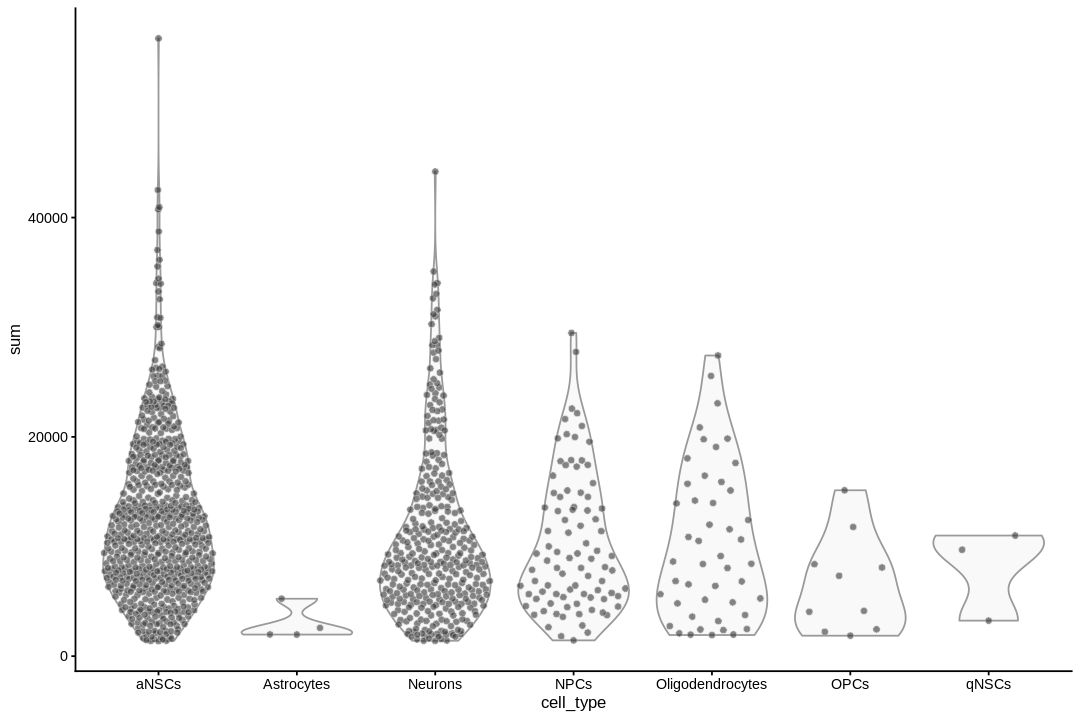`1` ``````plotColData(sce, x = "cell_type", y = "detected") ``````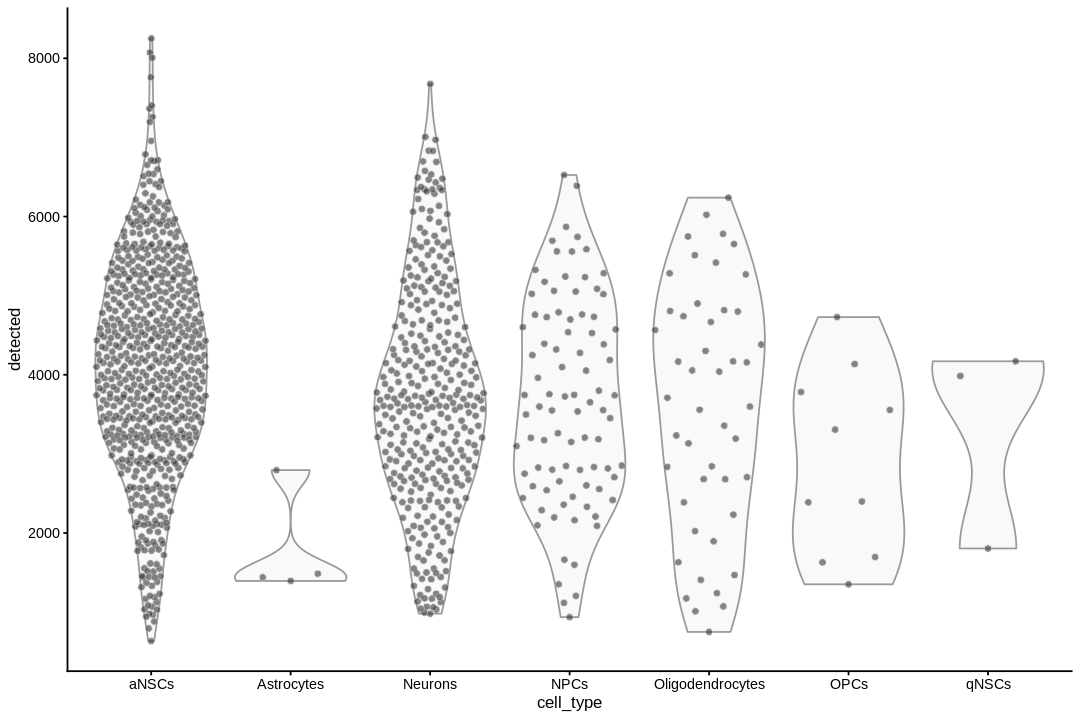```1 2 3 4``` ``````plotColData(sce, x = "sum", y = "detected", colour_by = "cell_type") + scale_x_log10() + scale_y_log10() + annotation_logticks() ``````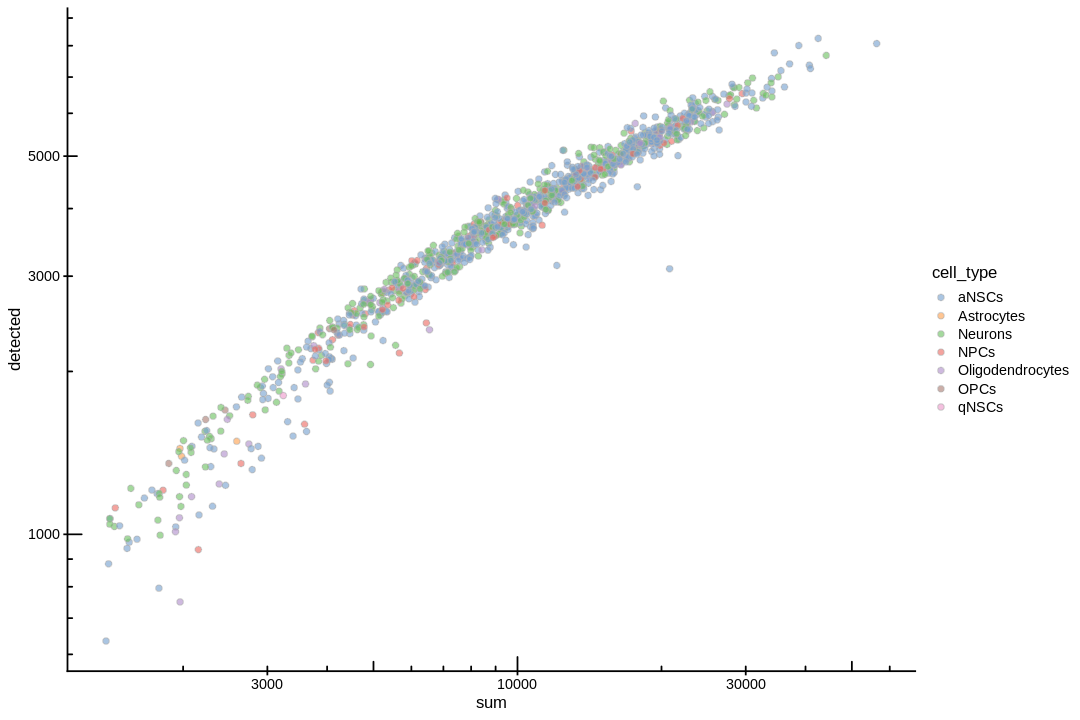## Dimension reduction¶

 ```1 2``` ``````dec_sce <- modelGeneVar(sce) fit_pbmc <- metadata(dec_sce) ``````
 ```1 2 3 4``` ``````# Adapted from https://osca.bioconductor.org/feature-selection.html#feature-selection plot(fit_pbmc\$mean, fit_pbmc\$var, xlab="Mean of log-expression", ylab="Variance of log-expression") curve(fit_pbmc\$trend(x), col="dodgerblue", add=TRUE, lwd=2) ``````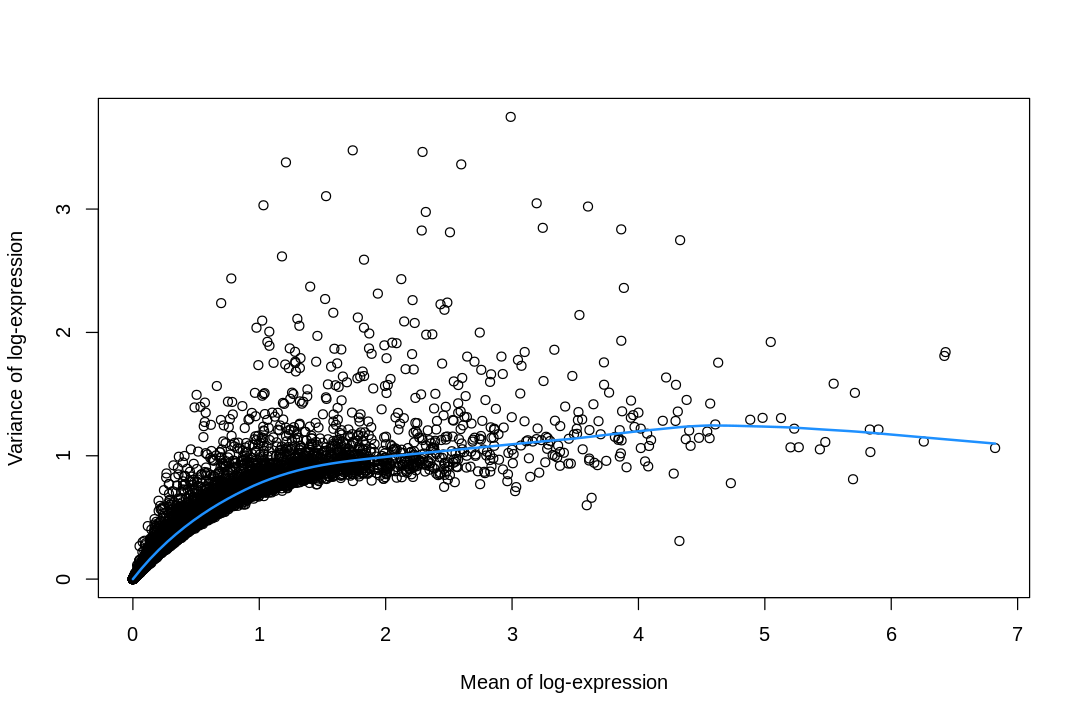```1 2``` ``````hvgs <- getTopHVGs(dec_sce, n = 3000) sce <- runPCA(sce, subset_row = hvgs) ``````
 ```1 2 3``` ``````# Percentage of variance explained is tucked away in the attributes. percent.var <- attr(reducedDim(sce), "percentVar") plot(percent.var, xlab="PC", ylab="Variance explained (%)") ``````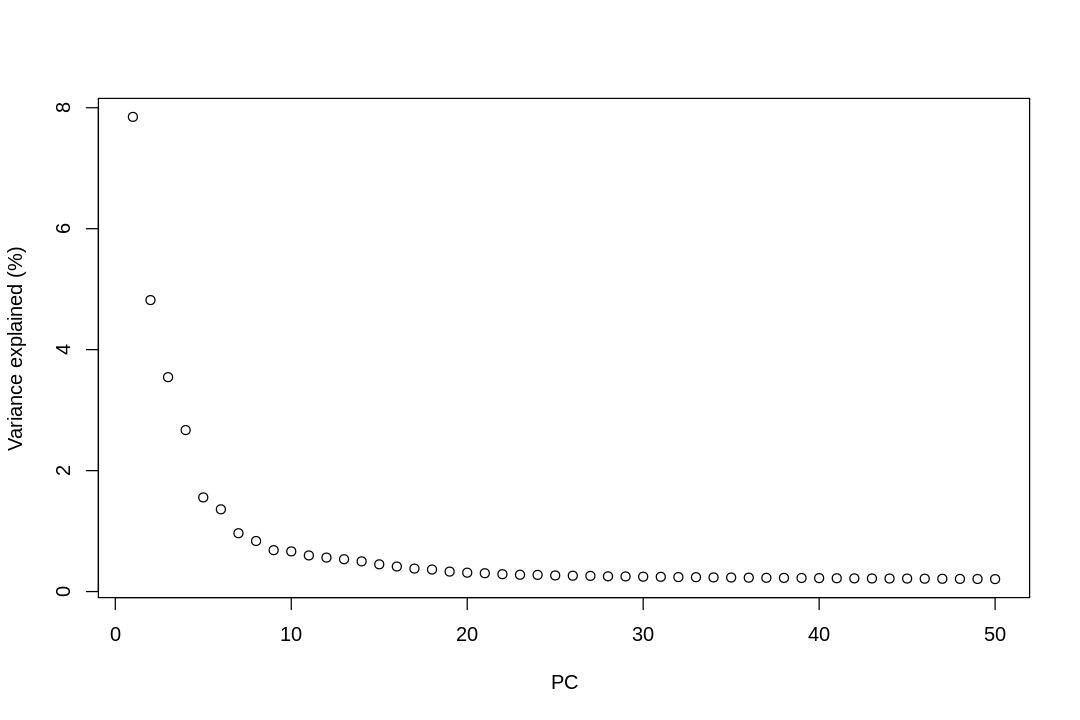The y axis is percentage of variance explained by the PC, or the eigenvalues of the covariance matrix.

 ```1 2``` ``````options(repr.plot.width=12, repr.plot.height=12) plotPCA(sce, colour_by = "cell_type", ncomponents = 4) `````````1 2 3``` ``````options(repr.plot.width=9, repr.plot.height=6) sce <- runTSNE(sce, dimred = "PCA") plotTSNE(sce, colour_by = "cell_type") `````````1 2 3``` ``````set.seed(1) sce <- runUMAP(sce, dimred = "PCA", n_neighbors = 30) plotUMAP(sce, colour_by = "cell_type") ``````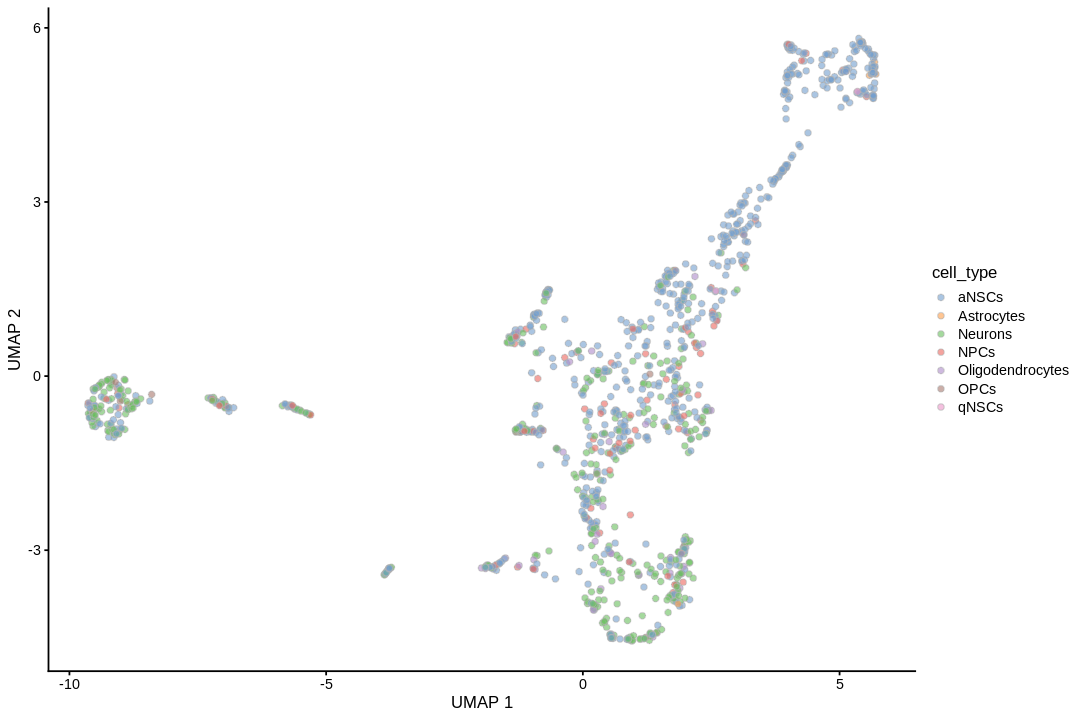UMAP can better preserve pairwise distance of cells than tSNE and can better separate cell populations than the first 2 PCs of PCA (Becht et al. 2018), so the TI will be visualized on UMAP rather than tSNE or PCA.

Cell type annotation with `SingleR` requires a reference with bulk RNA seq data for isolated known cell types. The reference used for cell type annotation here does not differentiate between different types of neural progenitor cells; clustering can further partition the neural progenitor cells. Furthermore, `slingshot` is based on cluster-wise minimum spanning tree, so finding a good clustering is important to good trajectory inference with `slingshot`. The clustering algorithm used here is Leiden, which is an improvement over the commonly used Louvain; Leiden communities are guaranteed to be well-connected, while Louvain can lead to poorly connected communities.

 `1` ``````g <- buildSNNGraph(sce, k = 15, use.dimred = "PCA") ``````
 ```1 2 3``` ``````sce\$cluster <- leidenbase::leiden_find_partition(g, resolution_parameter = 1.2, partition_type = "RBConfigurationVertexPartition", num_iter = 10, seed = 1)\$membership %>% factor() ``````
 `1` ``````plotUMAP(sce, colour_by = "cluster") ``````# Slingshot¶

## Trajectory inference¶

While the `slingshot` vignette uses `SingleCellExperiment`, `slingshot` can also take a matrix of cell embeddings in reduced dimension as input. We can optionally specify the cluster to start or end the trajectory based on biological knowledge. Here, since quiescent neural stem cells are in cluster 4, the starting cluster would be 4 near the top left of the previous plot.

I no longer consider doing trajectory inference on UMAP a good idea, due to distortions introduced by UMAP. See this paper for the extent non-linear dimension reduction methods distort the data. The latent dimension of the data is most likely far more than 2 or 3 dimensions, so forcing it down to 2 or 3 dimensions are bound to introduce distortions, just like how projecting the spherical surface of the Earth to 2 dimensions in maps introduces distortions. Furthermore, after the projection, some trajectories are no longer topologically feasible. For instance, imagine a stream coming out of the hole of a doughnut in 3D. This is not possible in 2D, so when that structure is projected to 2D, part of the stream may become buried in the middle of the doughnut, or the doughnut may be broken to allow the stream through, or part of the steam will be intermixed with part of the doughnut though they shouldn't. I recommend using a larger number of principal components instead, but in that case, the lineages and principal curves can't be visualized (we can plot the curves within a 2 dimensional subspace, such as the first 2 PCs, but that usually looks like abstract art and isn't informative about the lineages).

 ```1 2``` ``````sds <- slingshot(reducedDim(sce, "PCA")[,1:20], clusterLabels = sce\$cluster, start.clus = 2, stretch = 0) ``````
``````Using full covariance matrix
``````

Unfortunately, `slingshot` does not natively support `ggplot2`. So this is a function that assigns colors to each cell in base R graphics.

 ``` 1 2 3 4 5 6 7 8 9 10 11 12 13 14 15 16 17``` ``````#' Assign a color to each cell based on some value #' #' @param cell_vars Vector indicating the value of a variable associated with cells. #' @param pal_fun Palette function that returns a vector of hex colors, whose #' argument is the length of such a vector. #' @param ... Extra arguments for pal_fun. #' @return A vector of hex colors with one entry for each cell. cell_pal <- function(cell_vars, pal_fun,...) { if (is.numeric(cell_vars)) { pal <- pal_fun(100, ...) return(pal[cut(cell_vars, breaks = 100)]) } else { categories <- sort(unique(cell_vars)) pal <- setNames(pal_fun(length(categories), ...), categories) return(pal[cell_vars]) } } ``````

We need color palettes for both cell types and Leiden clusters. These would be the same colors seen in the Seurat plots.

 ```1 2``` ``````cell_colors <- cell_pal(sce\$cell_type, pal_d3(alpha = 0.5)) cell_colors_clust <- cell_pal(sce\$cluster, pal_d3(alpha = 0.5)) ``````
 ```1 2 3 4 5``` ``````par(mar=c(5.1, 4.1, 4.1, 14), xpd=TRUE) plot(reducedDim(sds, "PCA")[,1:2], col = cell_colors, pch = 16, cex = 1, xlab = "PC 1", ylab = "PC 2") lines(sds, lwd = 2, type = 'lineages', col = 'black') legend("right", inset = c(-0.6, 0), legend = unique(sce\$cell_type), col = unique(cell_colors), pch = 16, box.lwd = 0) ``````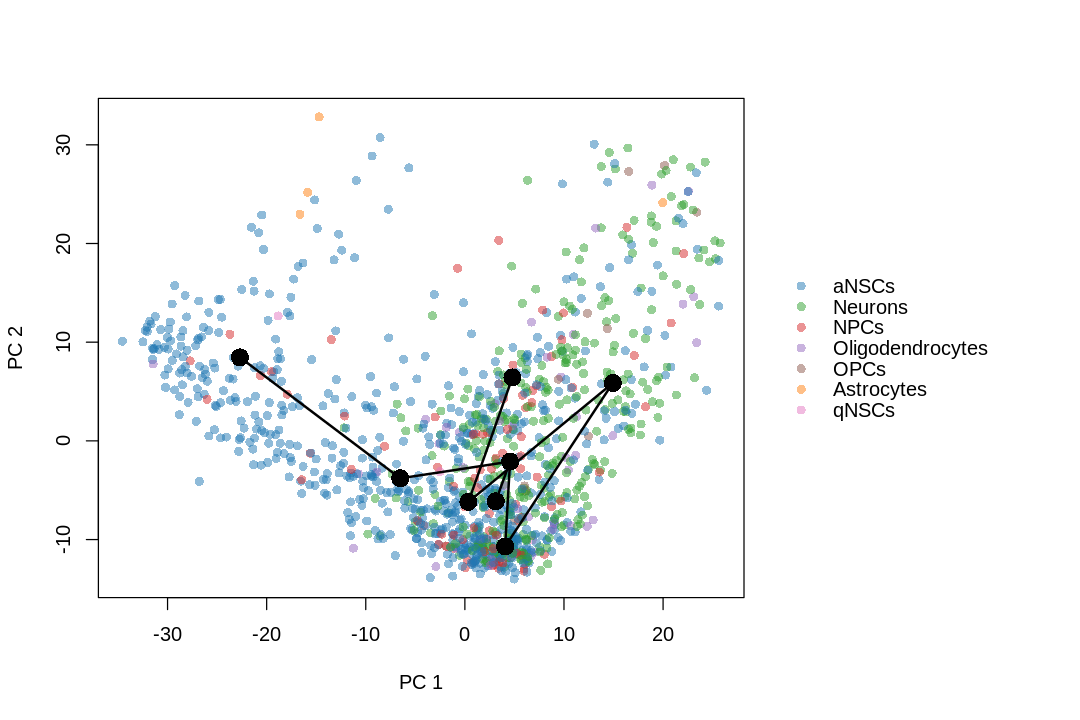```1 2 3 4 5 6``` ``````ord <- order(unique(sce\$cluster)) par(mar=c(5.1, 4.1, 4.1, 14), xpd=TRUE) plot(reducedDim(sds, "PCA")[,1:2], col = cell_colors_clust, pch = 16, cex = 1, xlab = "PC 1", ylab = "PC 2") lines(sds, lwd = 2, type = 'lineages', col = 'black') legend("right", inset = c(-0.2, 0), legend = unique(sce\$cluster)[ord], col = unique(cell_colors_clust)[ord], pch = 16, box.lwd = 0) ``````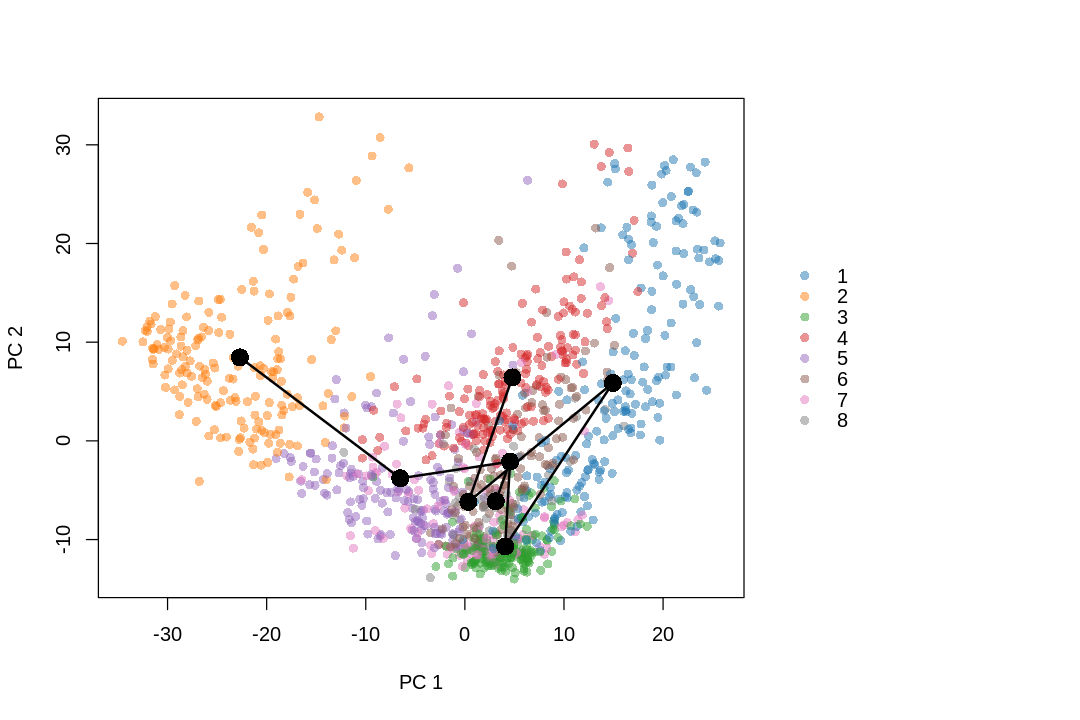```1 2 3 4 5``` ``````par(mar=c(5.1, 4.1, 4.1, 14), xpd=TRUE) plot(reducedDim(sds, "PCA")[,1:2], col = cell_colors, pch = 16, cex = 1, xlab = "PC 1", ylab = "PC 2") lines(sds, lwd = 2, col = 'black') legend("right", inset = c(-0.6, 0), legend = unique(sce\$cell_type), col = unique(cell_colors), pch = 16, box.lwd = 0) ``````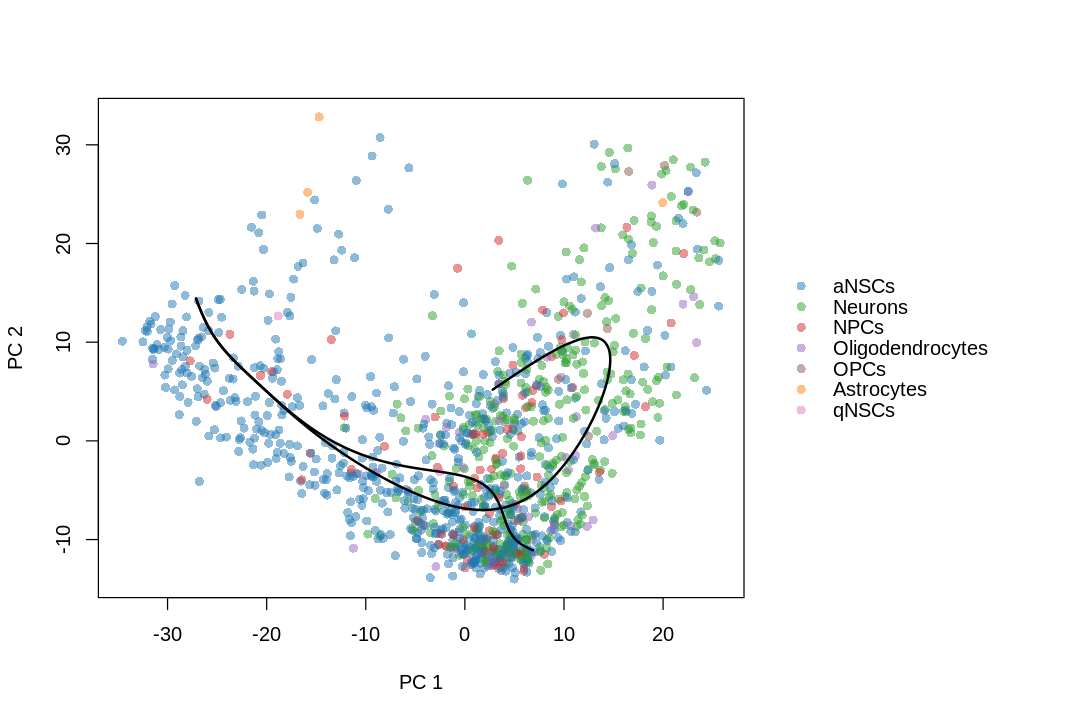Which cells are in which lineage? Here we plot the pseudotime values for each lineage.

 ``` 1 2 3 4 5 6 7 8 9 10 11 12``` ``````options(repr.plot.width=15, repr.plot.height=6) nc <- 3 pt <- slingPseudotime(sds) nms <- colnames(pt) nr <- ceiling(length(nms)/nc) pal <- viridis(100, end = 0.95) par(mfrow = c(nr, nc)) for (i in nms) { colors <- pal[cut(pt[,i], breaks = 100)] plot(reducedDim(sds), col = colors, pch = 16, main = i) lines(sds, lwd = 2, col = 'black', type = 'lineages') } ``````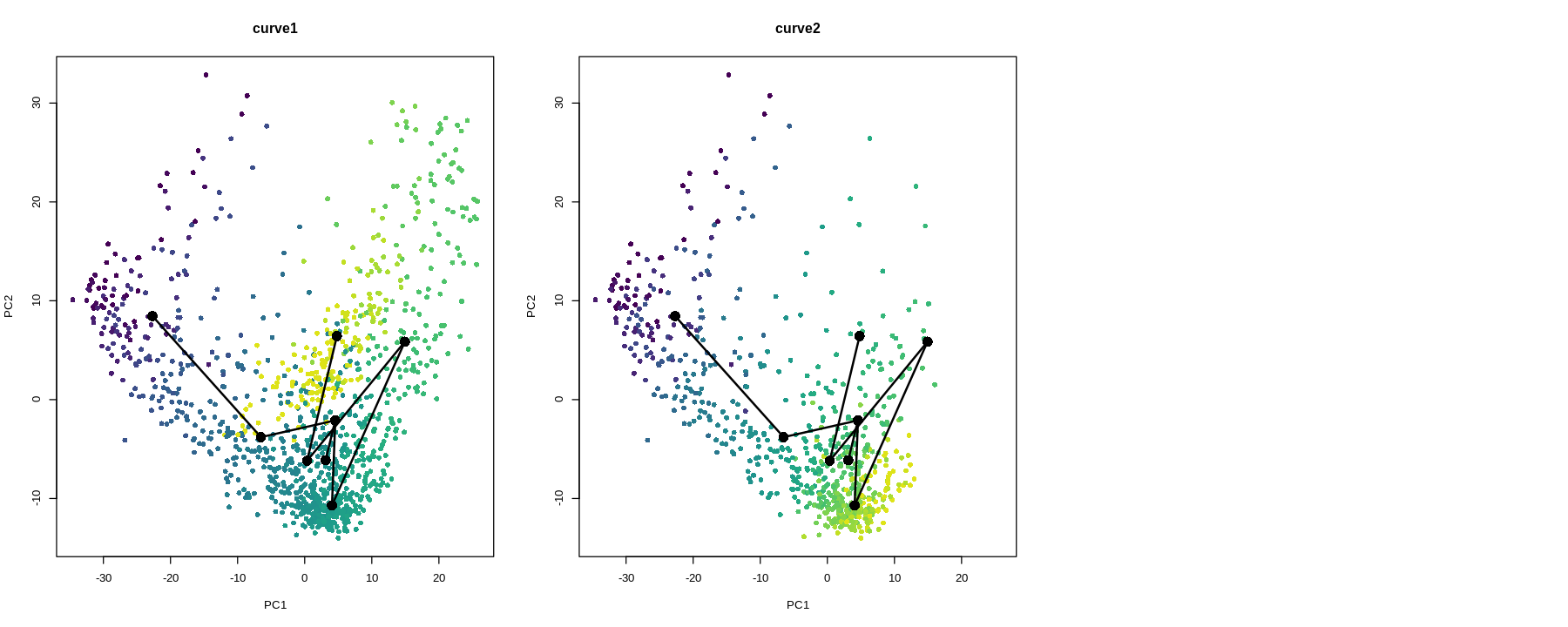Or on UMAP, though can't plot the lineages here

 ```1 2 3 4 5 6``` ``````par(mfrow = c(nr, nc)) options(repr.plot.width=15, repr.plot.height=6) for (i in nms) { colors <- pal[cut(pt[,i], breaks = 100)] plot(reducedDim(sce, "UMAP"), col = colors, pch = 16, main = i) } ``````# Differential expression¶

Here we use the package `tradeSeq`, presented in this paper. This section was adapted from the vignette of `tradeSeq`.

 ```1 2 3 4 5 6``` ``````control <- mgcv::gam.control() control\$maxit <- 1000 cts <- as.matrix(counts(sce)) icMat <- evaluateK(counts = cts, k=3:10, nGenes = 50, pseudotime = slingPseudotime(sds, na = FALSE), cellWeights = slingCurveWeights(sds), control = control) ``````
``````Warning message in newton(lsp = lsp, X = G\$X, y = G\$y, Eb = G\$Eb, UrS = G\$UrS, L = G\$L, :
"Fitting terminated with step failure - check results carefully"
Warning message in newton(lsp = lsp, X = G\$X, y = G\$y, Eb = G\$Eb, UrS = G\$UrS, L = G\$L, :
"Iteration limit reached without full convergence - check carefully"
Warning message in newton(lsp = lsp, X = G\$X, y = G\$y, Eb = G\$Eb, UrS = G\$UrS, L = G\$L, :
"Fitting terminated with step failure - check results carefully"
Warning message in newton(lsp = lsp, X = G\$X, y = G\$y, Eb = G\$Eb, UrS = G\$UrS, L = G\$L, :
"Fitting terminated with step failure - check results carefully"
Warning message in newton(lsp = lsp, X = G\$X, y = G\$y, Eb = G\$Eb, UrS = G\$UrS, L = G\$L, :
"Iteration limit reached without full convergence - check carefully"
Warning message in newton(lsp = lsp, X = G\$X, y = G\$y, Eb = G\$Eb, UrS = G\$UrS, L = G\$L, :
"Fitting terminated with step failure - check results carefully"
Warning message in newton(lsp = lsp, X = G\$X, y = G\$y, Eb = G\$Eb, UrS = G\$UrS, L = G\$L, :
"Fitting terminated with step failure - check results carefully"
Warning message in newton(lsp = lsp, X = G\$X, y = G\$y, Eb = G\$Eb, UrS = G\$UrS, L = G\$L, :
"Fitting terminated with step failure - check results carefully"
Warning message in newton(lsp = lsp, X = G\$X, y = G\$y, Eb = G\$Eb, UrS = G\$UrS, L = G\$L, :
"Fitting terminated with step failure - check results carefully"
Warning message in newton(lsp = lsp, X = G\$X, y = G\$y, Eb = G\$Eb, UrS = G\$UrS, L = G\$L, :
"Fitting terminated with step failure - check results carefully"
Warning message in newton(lsp = lsp, X = G\$X, y = G\$y, Eb = G\$Eb, UrS = G\$UrS, L = G\$L, :
"Fitting terminated with step failure - check results carefully"
Warning message in newton(lsp = lsp, X = G\$X, y = G\$y, Eb = G\$Eb, UrS = G\$UrS, L = G\$L, :
"Fitting terminated with step failure - check results carefully"
Warning message in newton(lsp = lsp, X = G\$X, y = G\$y, Eb = G\$Eb, UrS = G\$UrS, L = G\$L, :
"Fitting terminated with step failure - check results carefully"
Warning message in newton(lsp = lsp, X = G\$X, y = G\$y, Eb = G\$Eb, UrS = G\$UrS, L = G\$L, :
"Fitting terminated with step failure - check results carefully"
Warning message in newton(lsp = lsp, X = G\$X, y = G\$y, Eb = G\$Eb, UrS = G\$UrS, L = G\$L, :
"Iteration limit reached without full convergence - check carefully"
Warning message in newton(lsp = lsp, X = G\$X, y = G\$y, Eb = G\$Eb, UrS = G\$UrS, L = G\$L, :
"Fitting terminated with step failure - check results carefully"
Warning message in newton(lsp = lsp, X = G\$X, y = G\$y, Eb = G\$Eb, UrS = G\$UrS, L = G\$L, :
"Fitting terminated with step failure - check results carefully"
Warning message in newton(lsp = lsp, X = G\$X, y = G\$y, Eb = G\$Eb, UrS = G\$UrS, L = G\$L, :
"Fitting terminated with step failure - check results carefully"
Warning message in newton(lsp = lsp, X = G\$X, y = G\$y, Eb = G\$Eb, UrS = G\$UrS, L = G\$L, :
"Fitting terminated with step failure - check results carefully"
Warning message in newton(lsp = lsp, X = G\$X, y = G\$y, Eb = G\$Eb, UrS = G\$UrS, L = G\$L, :
"Iteration limit reached without full convergence - check carefully"
Warning message in newton(lsp = lsp, X = G\$X, y = G\$y, Eb = G\$Eb, UrS = G\$UrS, L = G\$L, :
"Iteration limit reached without full convergence - check carefully"
Warning message in newton(lsp = lsp, X = G\$X, y = G\$y, Eb = G\$Eb, UrS = G\$UrS, L = G\$L, :
"Iteration limit reached without full convergence - check carefully"
Warning message in newton(lsp = lsp, X = G\$X, y = G\$y, Eb = G\$Eb, UrS = G\$UrS, L = G\$L, :
"Iteration limit reached without full convergence - check carefully"
Warning message in newton(lsp = lsp, X = G\$X, y = G\$y, Eb = G\$Eb, UrS = G\$UrS, L = G\$L, :
"Iteration limit reached without full convergence - check carefully"
Warning message in newton(lsp = lsp, X = G\$X, y = G\$y, Eb = G\$Eb, UrS = G\$UrS, L = G\$L, :
"Fitting terminated with step failure - check results carefully"
Warning message in newton(lsp = lsp, X = G\$X, y = G\$y, Eb = G\$Eb, UrS = G\$UrS, L = G\$L, :
"Iteration limit reached without full convergence - check carefully"
Warning message in newton(lsp = lsp, X = G\$X, y = G\$y, Eb = G\$Eb, UrS = G\$UrS, L = G\$L, :
"Iteration limit reached without full convergence - check carefully"
Warning message in newton(lsp = lsp, X = G\$X, y = G\$y, Eb = G\$Eb, UrS = G\$UrS, L = G\$L, :
"Iteration limit reached without full convergence - check carefully"
Warning message in newton(lsp = lsp, X = G\$X, y = G\$y, Eb = G\$Eb, UrS = G\$UrS, L = G\$L, :
"Fitting terminated with step failure - check results carefully"
Warning message in newton(lsp = lsp, X = G\$X, y = G\$y, Eb = G\$Eb, UrS = G\$UrS, L = G\$L, :
"Fitting terminated with step failure - check results carefully"
Warning message in newton(lsp = lsp, X = G\$X, y = G\$y, Eb = G\$Eb, UrS = G\$UrS, L = G\$L, :
"Iteration limit reached without full convergence - check carefully"
Warning message in newton(lsp = lsp, X = G\$X, y = G\$y, Eb = G\$Eb, UrS = G\$UrS, L = G\$L, :
"Iteration limit reached without full convergence - check carefully"
Warning message in newton(lsp = lsp, X = G\$X, y = G\$y, Eb = G\$Eb, UrS = G\$UrS, L = G\$L, :
"Iteration limit reached without full convergence - check carefully"
Warning message in newton(lsp = lsp, X = G\$X, y = G\$y, Eb = G\$Eb, UrS = G\$UrS, L = G\$L, :
"Iteration limit reached without full convergence - check carefully"
Warning message in newton(lsp = lsp, X = G\$X, y = G\$y, Eb = G\$Eb, UrS = G\$UrS, L = G\$L, :
"Fitting terminated with step failure - check results carefully"
Warning message in newton(lsp = lsp, X = G\$X, y = G\$y, Eb = G\$Eb, UrS = G\$UrS, L = G\$L, :
"Iteration limit reached without full convergence - check carefully"
Warning message in newton(lsp = lsp, X = G\$X, y = G\$y, Eb = G\$Eb, UrS = G\$UrS, L = G\$L, :
"Iteration limit reached without full convergence - check carefully"
Warning message in newton(lsp = lsp, X = G\$X, y = G\$y, Eb = G\$Eb, UrS = G\$UrS, L = G\$L, :
"Iteration limit reached without full convergence - check carefully"
Warning message in newton(lsp = lsp, X = G\$X, y = G\$y, Eb = G\$Eb, UrS = G\$UrS, L = G\$L, :
"Iteration limit reached without full convergence - check carefully"
Warning message in newton(lsp = lsp, X = G\$X, y = G\$y, Eb = G\$Eb, UrS = G\$UrS, L = G\$L, :
"Iteration limit reached without full convergence - check carefully"
Warning message in newton(lsp = lsp, X = G\$X, y = G\$y, Eb = G\$Eb, UrS = G\$UrS, L = G\$L, :
"Iteration limit reached without full convergence - check carefully"
Warning message in newton(lsp = lsp, X = G\$X, y = G\$y, Eb = G\$Eb, UrS = G\$UrS, L = G\$L, :
"Iteration limit reached without full convergence - check carefully"
Warning message in newton(lsp = lsp, X = G\$X, y = G\$y, Eb = G\$Eb, UrS = G\$UrS, L = G\$L, :
"Iteration limit reached without full convergence - check carefully"
Warning message in newton(lsp = lsp, X = G\$X, y = G\$y, Eb = G\$Eb, UrS = G\$UrS, L = G\$L, :
"Iteration limit reached without full convergence - check carefully"
Warning message in newton(lsp = lsp, X = G\$X, y = G\$y, Eb = G\$Eb, UrS = G\$UrS, L = G\$L, :
"Iteration limit reached without full convergence - check carefully"
Warning message in newton(lsp = lsp, X = G\$X, y = G\$y, Eb = G\$Eb, UrS = G\$UrS, L = G\$L, :
"Iteration limit reached without full convergence - check carefully"
Warning message in newton(lsp = lsp, X = G\$X, y = G\$y, Eb = G\$Eb, UrS = G\$UrS, L = G\$L, :
"Iteration limit reached without full convergence - check carefully"
Warning message in newton(lsp = lsp, X = G\$X, y = G\$y, Eb = G\$Eb, UrS = G\$UrS, L = G\$L, :
"Iteration limit reached without full convergence - check carefully"
Warning message in newton(lsp = lsp, X = G\$X, y = G\$y, Eb = G\$Eb, UrS = G\$UrS, L = G\$L, :
"Iteration limit reached without full convergence - check carefully"
Warning message in newton(lsp = lsp, X = G\$X, y = G\$y, Eb = G\$Eb, UrS = G\$UrS, L = G\$L, :
"Iteration limit reached without full convergence - check carefully"
Warning message in newton(lsp = lsp, X = G\$X, y = G\$y, Eb = G\$Eb, UrS = G\$UrS, L = G\$L, :
"Fitting terminated with step failure - check results carefully"
Warning message in newton(lsp = lsp, X = G\$X, y = G\$y, Eb = G\$Eb, UrS = G\$UrS, L = G\$L, :
"Iteration limit reached without full convergence - check carefully"
Warning message in newton(lsp = lsp, X = G\$X, y = G\$y, Eb = G\$Eb, UrS = G\$UrS, L = G\$L, :
"Iteration limit reached without full convergence - check carefully"
Warning message in newton(lsp = lsp, X = G\$X, y = G\$y, Eb = G\$Eb, UrS = G\$UrS, L = G\$L, :
"Iteration limit reached without full convergence - check carefully"
Warning message in newton(lsp = lsp, X = G\$X, y = G\$y, Eb = G\$Eb, UrS = G\$UrS, L = G\$L, :
"Fitting terminated with step failure - check results carefully"
Warning message in newton(lsp = lsp, X = G\$X, y = G\$y, Eb = G\$Eb, UrS = G\$UrS, L = G\$L, :
"Iteration limit reached without full convergence - check carefully"
Warning message in newton(lsp = lsp, X = G\$X, y = G\$y, Eb = G\$Eb, UrS = G\$UrS, L = G\$L, :
"Iteration limit reached without full convergence - check carefully"
Warning message in newton(lsp = lsp, X = G\$X, y = G\$y, Eb = G\$Eb, UrS = G\$UrS, L = G\$L, :
"Iteration limit reached without full convergence - check carefully"
Warning message in newton(lsp = lsp, X = G\$X, y = G\$y, Eb = G\$Eb, UrS = G\$UrS, L = G\$L, :
"Iteration limit reached without full convergence - check carefully"
Warning message in newton(lsp = lsp, X = G\$X, y = G\$y, Eb = G\$Eb, UrS = G\$UrS, L = G\$L, :
"Fitting terminated with step failure - check results carefully"
Warning message in newton(lsp = lsp, X = G\$X, y = G\$y, Eb = G\$Eb, UrS = G\$UrS, L = G\$L, :
"Fitting terminated with step failure - check results carefully"
Warning message in newton(lsp = lsp, X = G\$X, y = G\$y, Eb = G\$Eb, UrS = G\$UrS, L = G\$L, :
"Fitting terminated with step failure - check results carefully"
Warning message in newton(lsp = lsp, X = G\$X, y = G\$y, Eb = G\$Eb, UrS = G\$UrS, L = G\$L, :
"Iteration limit reached without full convergence - check carefully"
Warning message in newton(lsp = lsp, X = G\$X, y = G\$y, Eb = G\$Eb, UrS = G\$UrS, L = G\$L, :
"Iteration limit reached without full convergence - check carefully"
``````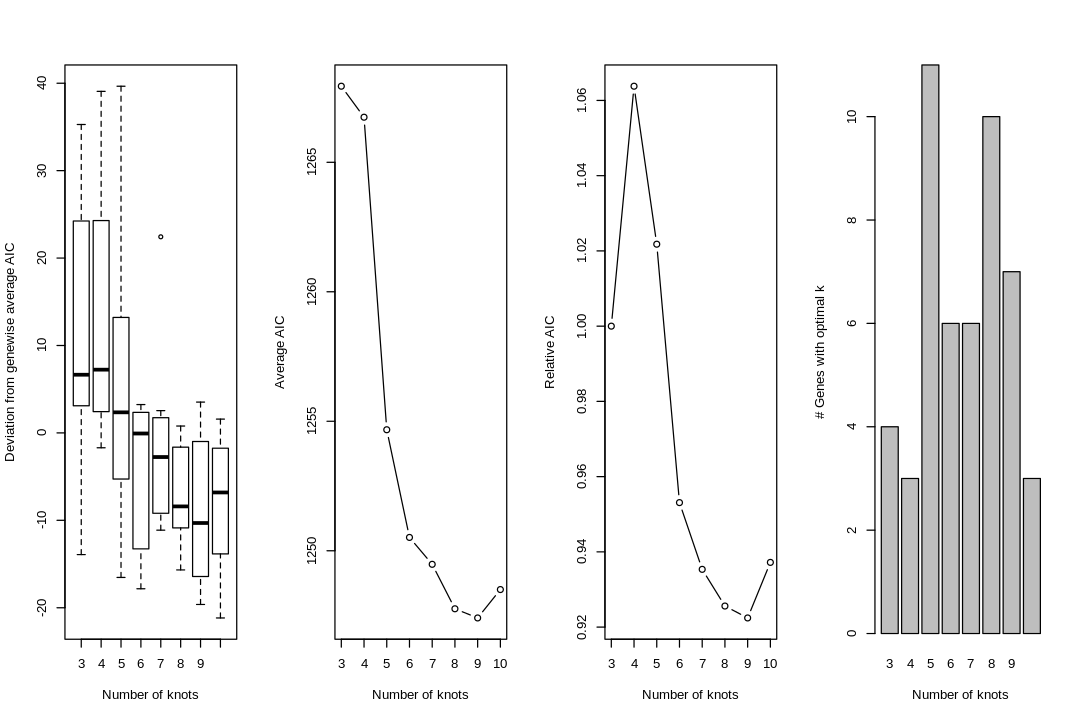```1 2``` ``````register(MulticoreParam(2)) sce2 <- fitGAM(counts = cts[hvgs[1:50],], sds = sds, nknots = 5, control = control) ``````

See where the knots are

 ```1 2``` ``````plotGeneCount(curve = sds, clusters = sce\$cluster, models = sce2) ``````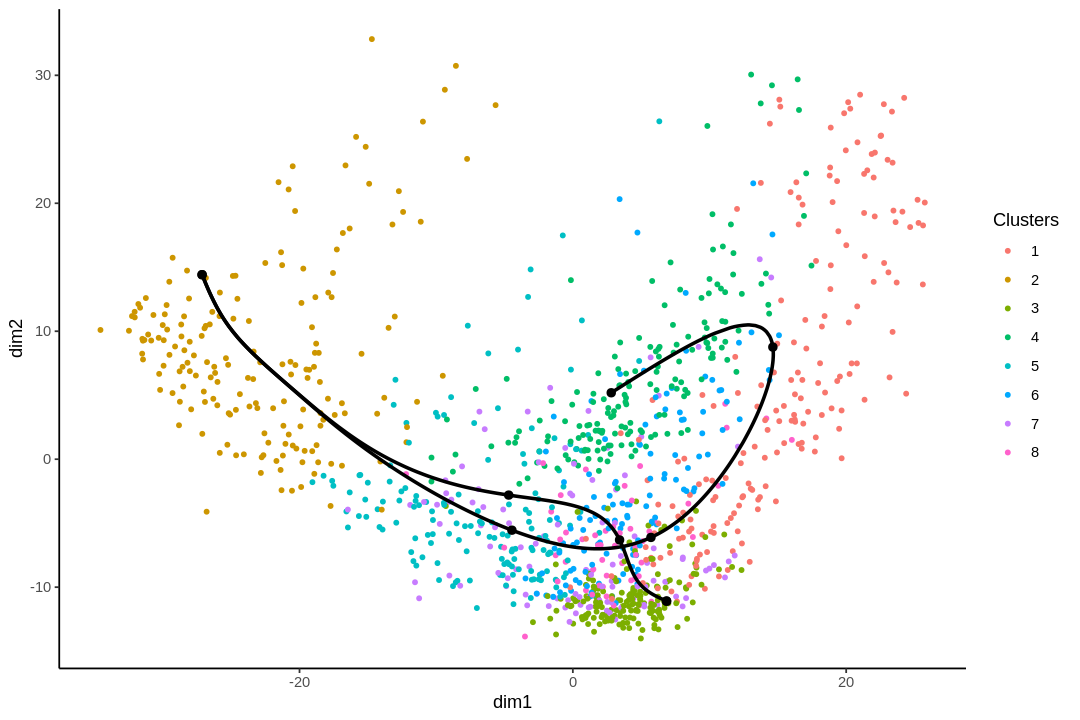## Within linenage comparisons¶

 ```1 2``` ``````assoRes <- associationTest(sce2) head(assoRes) ``````
A data.frame: 6 × 3
waldStatdfpvalue
<dbl><dbl><dbl>
ENSMUSG00000027500 749.620970
ENSMUSG000000198741765.895570
ENSMUSG00000005583 579.496670
ENSMUSG00000029838 504.341570
ENSMUSG00000027210 492.377470
ENSMUSG000000267281072.927570
 `1` ``````startRes <- startVsEndTest(sce2) ``````
 ```1 2 3``` ``````oStart <- order(startRes\$waldStat, decreasing = TRUE) sigGeneStart <- names(sce2)[oStart] plotSmoothers(sce2, cts, gene = sigGeneStart) ``````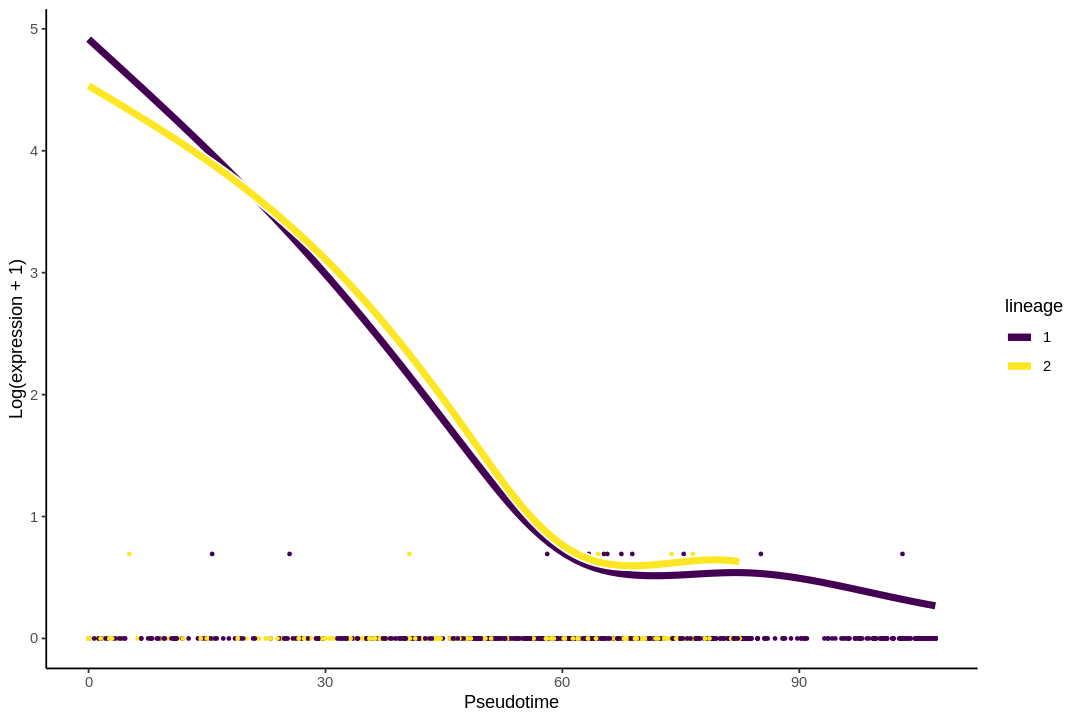`1` ``````plotUMAP(sce, colour_by = sigGeneStart) ``````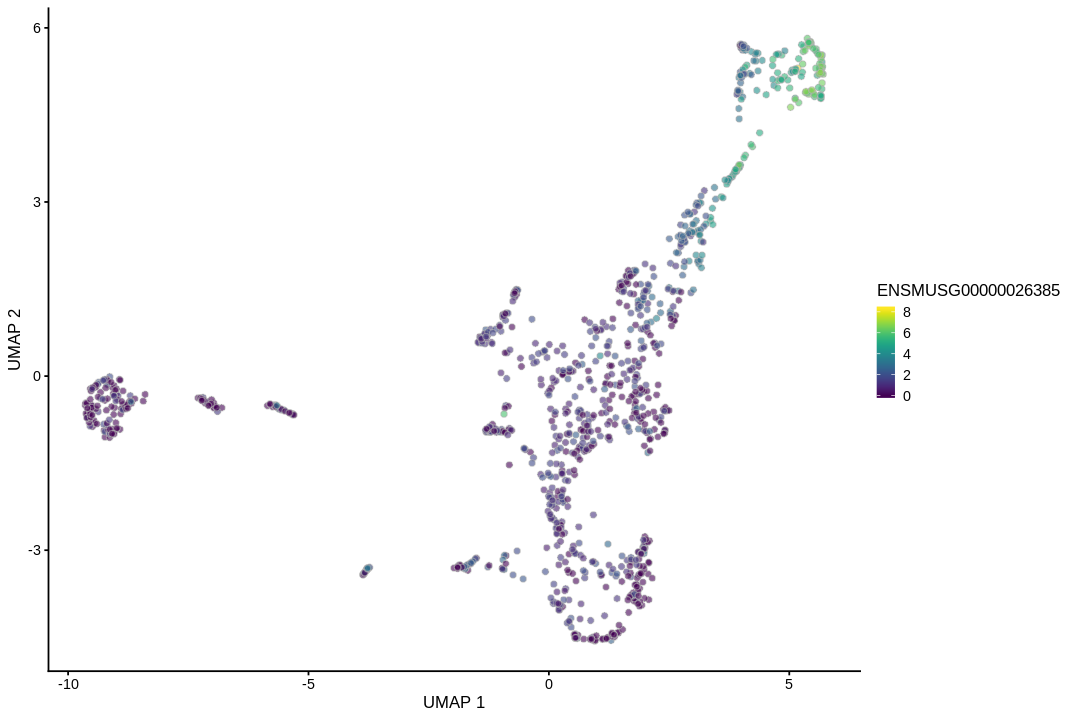## Between lineage comparisons¶

 `1` ``````endRes <- diffEndTest(sce2) ``````
 ```1 2 3``` ``````o <- order(endRes\$waldStat, decreasing = TRUE) sigGene <- names(sce2)[o] plotSmoothers(sce2, cts, sigGene) ``````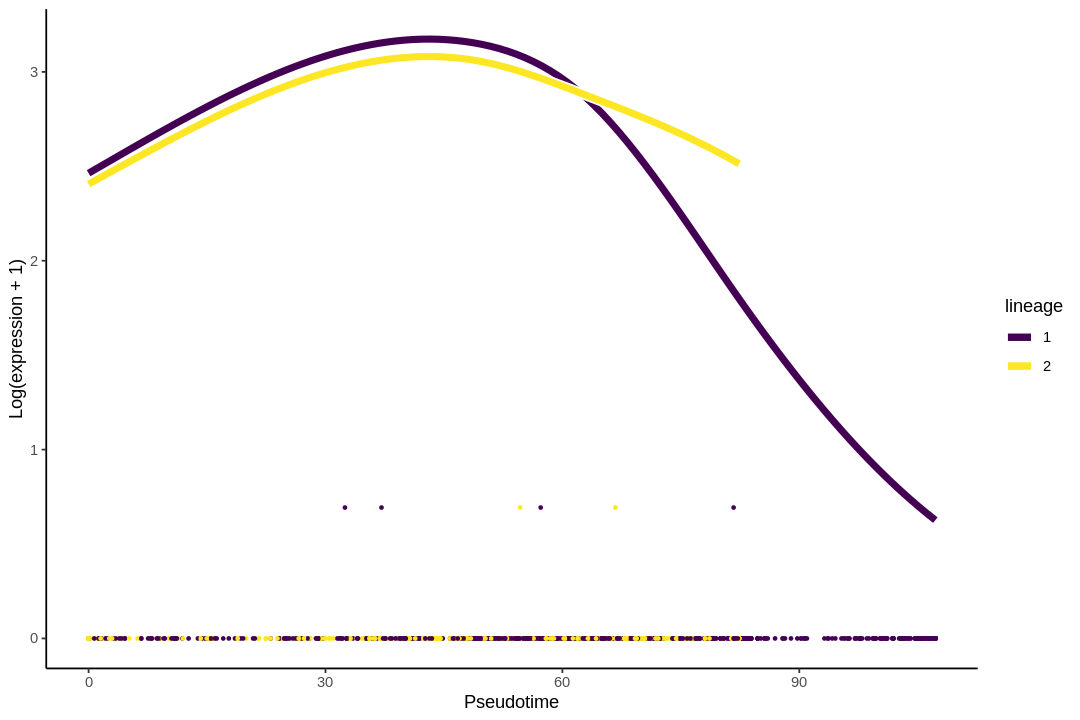`1` ``````plotUMAP(sce, colour_by = sigGene) ``````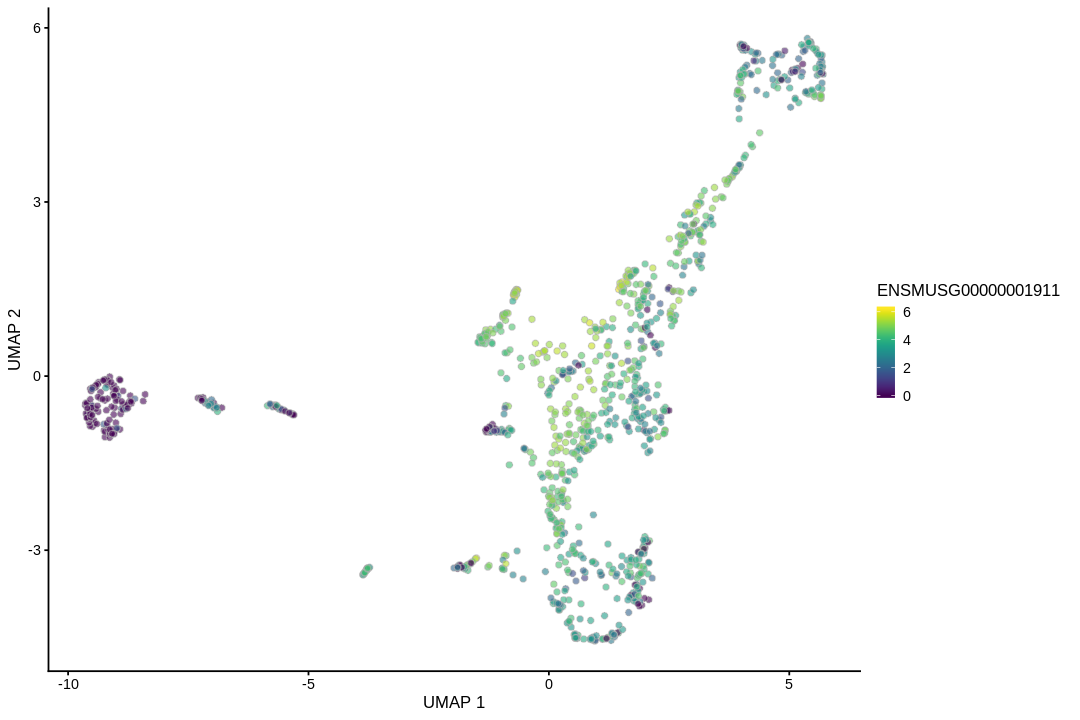## Different patterns¶

 ```1 2 3``` ``````patternRes <- patternTest(sce2) oPat <- order(patternRes\$waldStat, decreasing = TRUE) head(rownames(patternRes)[oPat]) ``````
1. 'ENSMUSG00000035551'
2. 'ENSMUSG00000027239'
3. 'ENSMUSG00000018411'
4. 'ENSMUSG00000067786'
5. 'ENSMUSG00000052727'
6. 'ENSMUSG00000058672'
 `1` ``````plotSmoothers(sce2, cts, gene = rownames(patternRes)[oPat]) ``````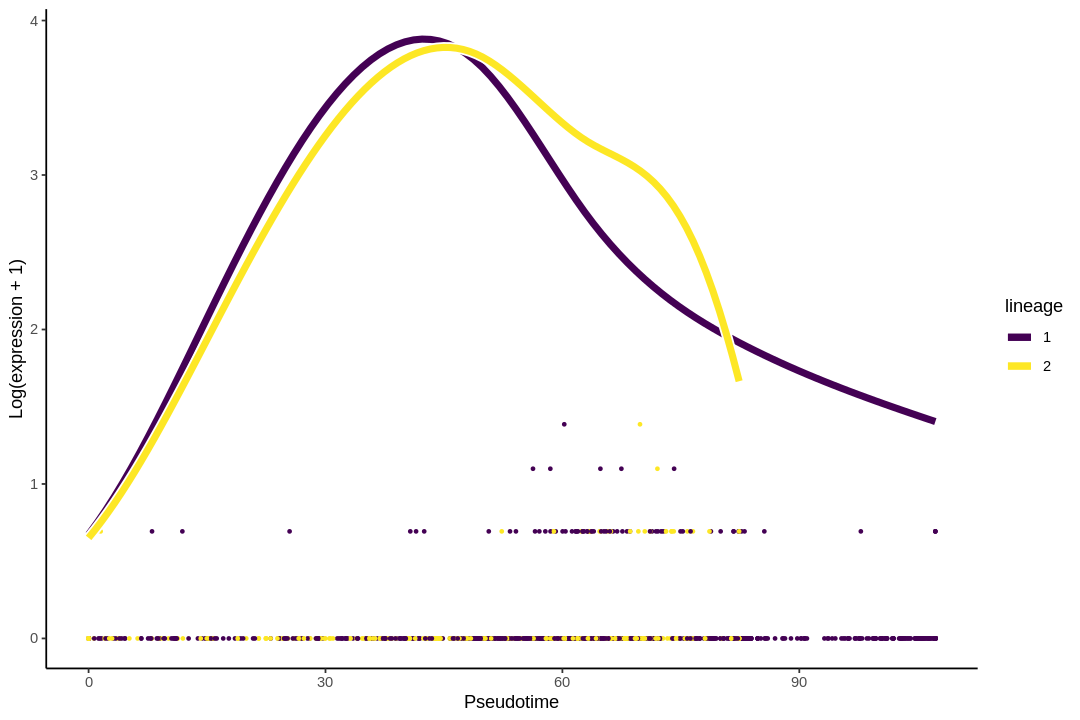`1` ``````plotUMAP(sce, colour_by = rownames(patternRes)[oPat]) ``````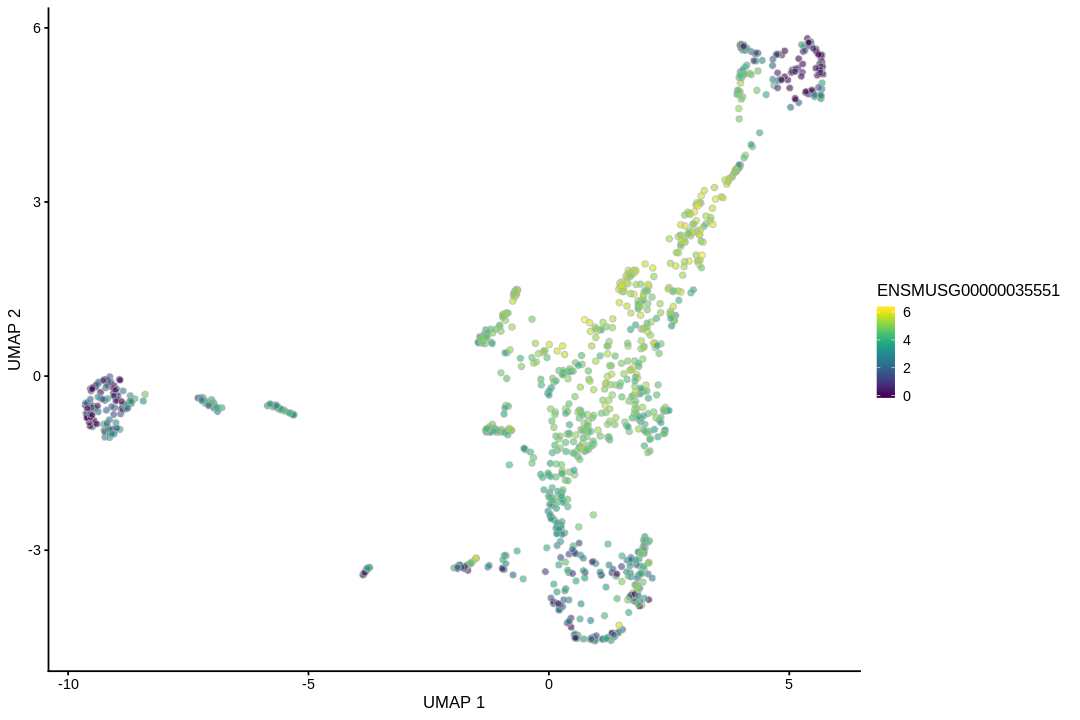## Clustering patterns¶

 ```1 2 3``` ``````nPointsClus <- 10 clusPat <- clusterExpressionPatterns(sce2, nPoints = nPointsClus, genes = rownames(sce2)) ``````
 `1` ``````clusterLabels <- primaryCluster(clusPat\$rsec) ``````
 ``` 1 2 3 4 5 6 7 8 9 10 11 12 13 14 15 16 17 18 19 20 21 22 23 24 25 26 27 28``` ``````cUniq <- unique(clusterLabels) cUniq <- cUniq[!cUniq == -1] # remove unclustered genes plots <- list() for (xx in cUniq[1:4]) { cId <- which(clusterLabels == xx) p <- ggplot(data = data.frame(x = 1:nPointsClus, y = rep(range(clusPat\$yhatScaled[cId, ]), nPointsClus / 2)), aes(x = x, y = y)) + geom_point(alpha = 0) + labs(title = paste0("Cluster ", xx), x = "Pseudotime", y = "Normalized expression") + theme_classic() for (ii in 1:length(cId)) { geneId <- rownames(clusPat\$yhatScaled)[cId[ii]] p <- p + geom_line(data = data.frame(x = rep(1:nPointsClus, 2), y = clusPat\$yhatScaled[geneId, ], lineage = rep(0:1, each = nPointsClus)), aes(col = as.character(lineage), group = lineage), lwd = 1.5) } p <- p + guides(color = FALSE) + scale_color_manual(values = c("orange", "darkseagreen3"), breaks = c("0", "1")) plots[[as.character(xx)]] <- p } plots\$ncol <- 2 do.call(cowplot::plot_grid, plots) ``````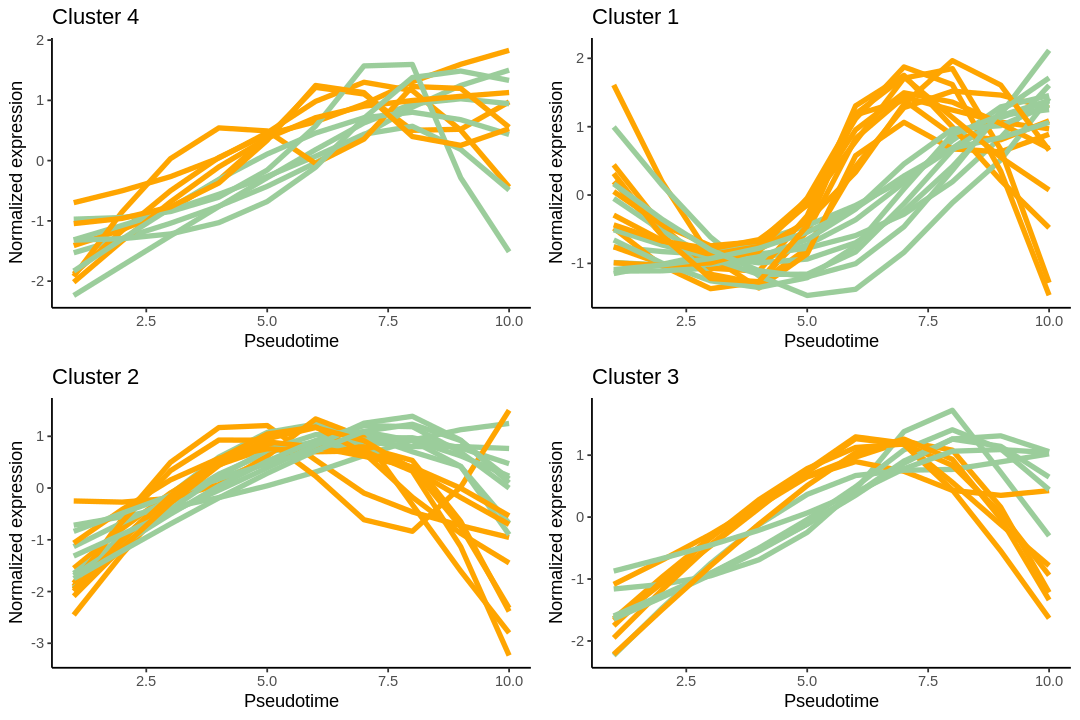`1` `````` ``````